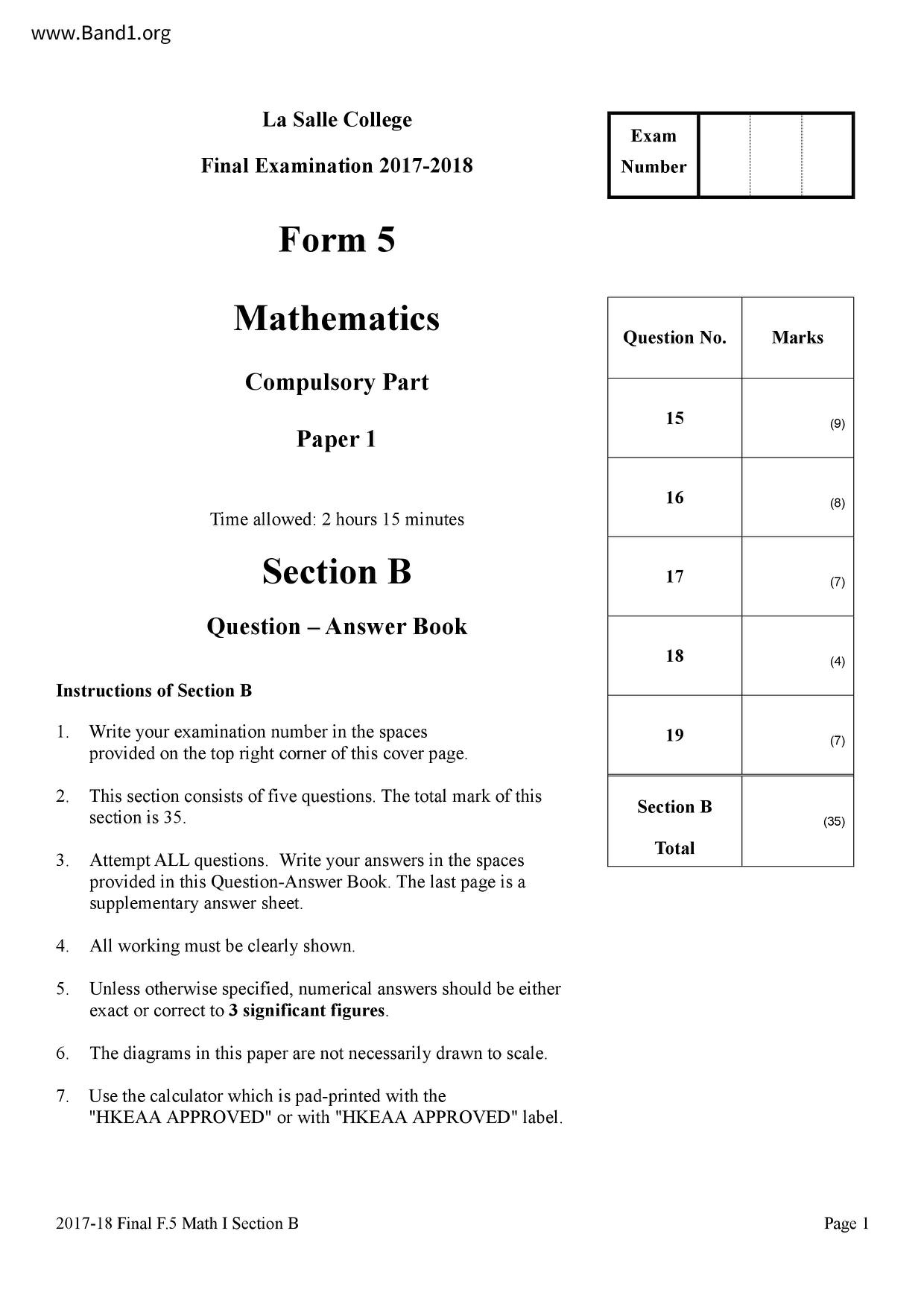# 中五 數學試卷 (F5 Maths Past Paper)

6628

pdf

12

718_F5_FINAL_MATHS_CP_1B

▼ 圖片只作預覽, 如欲下載整份卷, 請按「免費成為會員」 ▼▲ 圖片只作預覽, 如欲下載整份卷, 請按「免費成為會員」 ▲

## 中五數學試卷 PDF 下載

Instructions of Section B
La Salle College
Final Examination 2017-2018
Mathematics
Compulsory Part
Time allowed: 2 hours 15 minutes
provided in this Question-Answer Book. The last page is a
All working must be clearly shown.
Unless otherwise specified, numerical answers should be either
exact or correct to 3 significant figures.
The diagrams in this paper are not necessarily drawn to scale.
Use the calculator which is pad-printed with the
"HKEAA APPROVED" or with "HKEAA APPROVED" label.
Write your examination number in the spaces
provided on the top right corner of this cover page.
This section consists of five questions. The total mark of this
section is 35.
2017-18 Final F.5 Math I Section B
Question No.
2017-18 Final F.5 Math I Section B
Fifteen dots are evenly spaced on the circumference of a circle.
(a) Tim claims that it is impossible to form a right angled triangle by picking 3 dots from these 15
(b) How many combinations of three dots can we pick from the 15 dots so that an isosceles triangle
can be formed?
(c) How many combinations of three dots can we pick from the 15 dots so that an acute angled
triangle can be formed?
2017-18 Final F.5 Math I Section B
End of Section B
Question No.:
2017-18 Final F.5 Math I Section B
Section B (35 marks)
15. In the figure, ABCD is a circle. AB and DC are produced to meet at E. It is given that AC = CE and B
is the mid-point of AE.
A rectangular coordinate system is introduced in the figure so that DE is parallel to the x-axis
and the coordinates of A are (-1, 0). It is given that the equation of the circle is
y²- 6x6y-7= 0. Find
2017-18 Final F.5 Math I Section B
(i) the coordinates of C,
(ii) the equation of the straight line passing through B and C.
2017-18 Final F.5 Math I Section B
It is given that f(x) is the sum of two parts, one part varies as x² and the other part varies as x.
Suppose that f(1) = −25 and ƒ(7) = 35.
Solve f(x) = -40.
A(a, -40), B(b, -40) and C(c, 0) are points on the graph of y = f(x), where c = 0.
Find the area of the quadrilateral OABC, where O is the origin.
2017-18 Final F.5 Math I Section B
2017-18 Final F.5 Math I Section B
The stem-and-leaf diagram below shows the distribution of the weekly working time (in hours) of
employees in a company.
Stem (tens)
Leaf (units)
2017-18 Final F.5 Math I Section B
It is given that the mean and the range of the above distribution are 41 and 29 respectively.
Find the standard deviation of the above distribution.
Janice is one of the employees in the company.
A new employee is joined to the company whose weekly working time is 41 hours.
Explain briefly how Janice's standard score will change.
2017-18 Final F.5 Math I Section B
Ada invites John and eight other friends to join a party. All have to bring along with a present for
exchange. Each participant will draw a present randomly without replacement.
(a) Find the probability that John will get his own present but Ada will not.
Given that Ada does not get her own present, find the probability that John will get his own
2017-18 Final F.5 Math I Section B
2017-18 Final F.5 Math I Section B